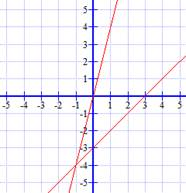### Sample Problem

Solve the following system of equations by graphing

y = x – 3

y = 4x(, )

#### Solution

Graph the system of equations:

y = x – 3

y = 4xWe can see from the graph that the two lines intersect at (-1, -4), so (-1, -4) is the solution to this system of equations.